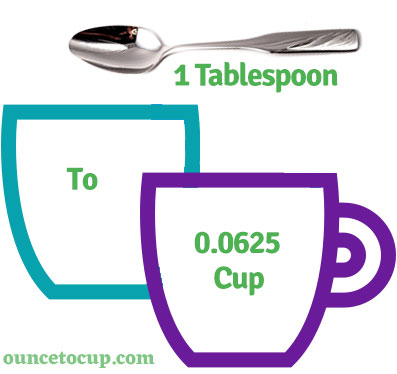# Tablespoons to Cups (tbsp to c conversion)

Are you cooking your favorite dish? The detailed chart in the recipe includes the calculation of the 1 tablespoon to cup conversion.  Don't worry; use this calculator to determine how many 1 tablespoon equals cups in a minute.  This 1 tbsp to c converter gives an exact measurement for any recipe you prepare.

Tablespoon Value:

tbsp

Cup Value:

c

1 Tablespoon = 0.0625 Cup
(1 tbsp = 0.0625 c)

Try our auto 1 Tablespoon to Cup Calculator (Without Convert Button), Just change the first field value and you got final value.
Tablespoon [tbsp]Cup [c]
1 Tablespoon1/6 Cup
2 Tablespoon1/8 Cup
4 Tablespoon1/4 Cup
5 Tablespoon1/3 Cup
8 Tablespoon1/2 Cup
11 Tablespoon2/3 Cup
12 Tablespoon3/4 Cup
16 Tablespoon1 Cup## How many cup is a tbsp?

We know that the mass value of 1 tbsp is equal to 0.0625 c. If you want to convert 1 tbsp to an equal number of cup, just divide the volume value by 16. Hence, 1 tablespoon is equal to 0.0625 cup.

The Answer is: 1 US Tablespoons = 0.0625 US Cup

1 tbsp = 0.0625 c

Many of them try to search or find an answer for what is 1 tablespoons in c? So, we’ll start with 1 tbsp to c conversion to know how big is 1 tbsp.

## How To Calculate 1 tbsp to cup?

To calculate 1 tablespoons to an equal number of cup, simply follow the steps below.

Tablespoons to Cups formula is:

Cup = Tablespoon / 16

Assume that we are finding out how many cup were found in 1 tbsp, divide by 16 to get the result.

Applying to Formula: cup = 1 tbsp / 16 = 0.0625 c.

## How To Convert tbsp to cup?

• To convert tablespoons to cup,
• Simply divide the tablespoon value by 16.
• Applying to the formula, cup = 1 tablespoons / 16 [1/16].
• Hence, 1 tablespoons is equal to 0.0625 cup.
Tablespoons [tbsp]Cups [c]
1 tbps0.0625 cup
2 tbps0.125 cup
3 tbps0.1875 cup
4 tbps0.25 cup
5 tbps0.3125 cup
6 tbps0.375 cup
7 tbps0.4375 cup
8 tbps0.5 cup
9 tbps0.5625 cup
10 tbps0.625 cup
11 tbps0.6875 cup
12 tbps0.75 cup
13 tbps0.8125 cup
14 tbps0.875 cup
15 tbps0.9375 cup
16 tbps1 cup

## Reverse Calculation: How many tablespoons are in a cup?

• To convert 1 cup to tbsp,
• Simply multiply the 1 cup by 16.
• Then, applying the formula, tbsp = 1 cup * 16 [1x16 = 16].
• Hence, 1 cup is equal to 16 tablespoon.

### Related Converter:

Formula: tablespoon to cup

cup = tablespoon / 0.0625

Applying to Formula,

c = 1/16 = 0.0625

1 tablespoon = 0.0625 cup